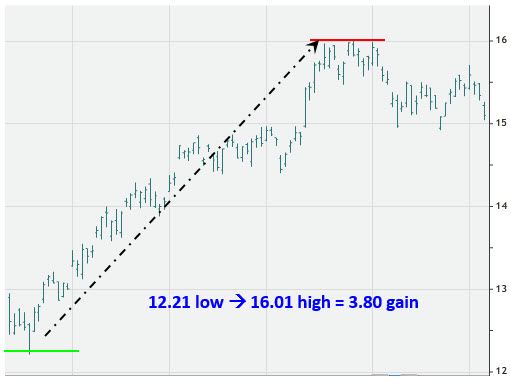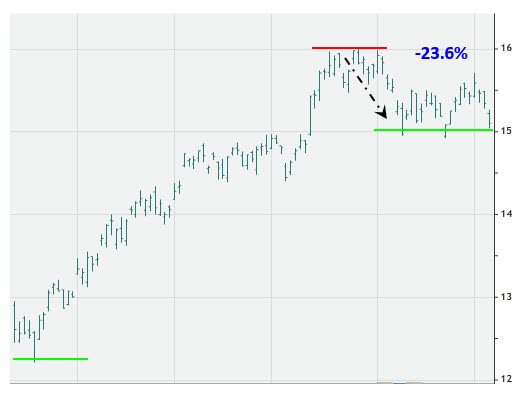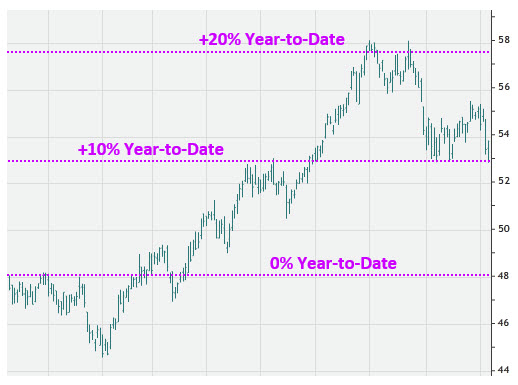# What You Need to Know About Fibonacci Levels

## Every trader should be aware of the impact Fibonacci levels and round-number percentage returns can have on stocks

Dec 15, 2015 at 3:26 PM

Whether you're trading stocks or options, you probably include technical analysis somewhere in your methodology. The next time you analyze a chart, remember that there are two types of percentage returns that all traders should have on their radar:

1. Fibonacci levels
2. Round-number percentages
You may already be familiar with Fibonacci numbers. Essentially, it's a sequence of numbers developed by the medieval Italian mathematician Leonardo Fibonacci, where each successive integer represents the sum of the two numbers preceding it.

Each number in the Fibonacci sequence -- 1, 1, 2, 3, 5, 8, 13, 21, 34, 55, 89, 144, and so on -- is roughly 1.618 times greater than the number directly before it. For example, 13 x 1.618 = 21.034.

The Fibonacci retracement levels used in technical analysis are derived by dividing one of these numbers by another number that appears later in the chain. For example, 55 divided by 89 is 61.8% -- known as the "golden ratio." Moving two numbers to the right in the sequence, 55 divided by 144 is 38.2%.

Other key Fibonacci retracement levels include 23.6%, which results from dividing a Fibonacci number by the integer three places to the right, and 76.4%, which is found by subtracting 23.6 from 100. Additionally, 100% and 50% are also considered Fibonacci retracement levels, since 1 divided by 1 is 100%, and 1 divided by 2 is 50%.

These are the key Fibonacci retracement levels used by technical analysts to determine potential areas of support or resistance.

• 23.6%
• 38.2%
• 50%
• 61.8%
• 76.4%
• 100%
To find Fibonacci retracement levels, subtract the stock's high price from its low price. Retracement levels are based on the difference between the two numbers.For example, a stock may find support after retracing 23.6% of a recent rally. If a stock pulls back to one of these Fibonacci retracement levels and turns higher, it could signal an opportune entry point for a bullish call trade.In addition to Fibonacci levels, stocks will often find support at price points that correspond with round-number percentage returns. For example, after declining 20% off a recent high, a stock may look more affordable, and traders who were waiting for a dip may decide to buy.

We've witnessed this supportive phenomenon play out with a variety of round-number levels, such as 10%, 20%, 50%, and even 80%.

It's also worth watching percentage returns from year-to-date levels, or from other significant price points -- such as all-time highs and lows, or initial public offering prices.Of course, the flip side of this phenomenon is that stocks have been known to encounter resistance after gaining 10%, 20%, or 50% from a meaningful price point. Traders should be aware of these areas as potential sticking points before buying a call.

All charts courtesy of OptionsHouse.

Grab your FREE Eternal Contrarian report!